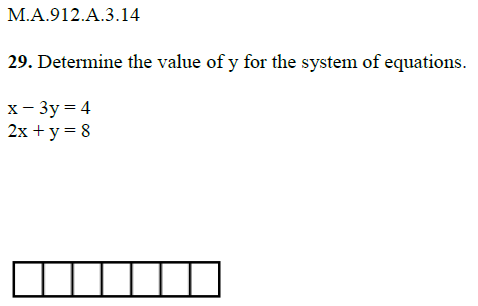Question 29: Finding the Value of y

Algebra Level 1MA.912.A.3.14 - Solve systems of linear equations and inequalities in two and three variables using graphical, substitution, and elimination methods.

Algebra 1 EOC Study Guide. How many can you solve correctly?

×